Home > Excel > Top 7 Reasons and Solutions for 'Excel Formula Not Working' Issue

# Top 7 Reasons and Solutions for 'Excel Formula Not Working' Issue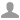Ronnie Barnes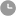Last updated: Mar 20, 2023 12:00 am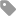Excel

Microsoft Excel is a powerful tool to create and store valuable information and databases about different features or aspects. Features not only include calculations, pivot tables and pivot graph but also supports a macro programming language known as Visual Basic for applications. MS excel is broadly used as a standard tool for data creation, visualization and data analysis. To become highly proficient in Microsoft Excel for financial analysis, learning and mastering the Excel formulas is essential for any user.

MS Excel's spreadsheet is also used by financial data analysts and investment bankers for the presentation of data insights, visualizations and financial modeling. But sometimes you may run into trouble even using such highly sophisticated piece of software tool. Can you imagine if you are working in hard deadlines and you suddenly realize that your excel formula is not working properly? Of course, It is a chaos, but don't worry we are here to help you out if your "Excel formula is not working".

Any user of MS excel may run into similar issues that could cause immense frustration, especially in the case when all of sudden Excel formulas stop working. While dealing with excel formulas, some major issues that you may face are: Excel formula is not calculating the value or Excel formula is not updating the value automatically. If you are facing similar issues, you do not need to worry anymore as you are going to resolve the issues in a moment and will get all the answer for your questions that are in your mind.

## Part 1. Basic Calculations and Terms in MS Excel

You may skip this section and move to the next section if you are not a beginner in Microsoft Excel and know well about the formulas and functions of MS Excel.

To perform any calculation in your Excel sheet, there are two basic ways that you can use i.e. Functions and Formulas.

Function: A function is nothing more than a predefined formula in MS Excel. These are already defined in the program to facilitate you and eliminate the factor of entering manual formulas for your data. These predefined formulas have user-friendly names such as sum, count, average and finding maximum value or minimum value for a range of cells. "AVERAGE" is a function that is used to calculate the average for the elements in a given range of the cells. For Example, if you apply the function "=AVERAGE(C2:C7)", It would calculate the average values of the elements in the cells ranging from C2 to C7. "=AVERAGE(C2:C7)" contains a single argument, bit if you want to pass multiple arguments they must be separated by commas. For Example, "=AVERAGE(C2:C7, B1:B4, D3:D9)" will calculate the average value of all cell ranges in three arguments.

Formula: A formula on the other hand is a user-defined expression in Excel. It performs the specified operation on a cell or on values in range of cells. For Example, the expression "=D2+D23+D4" will find the sum of the range of the values that are from cell D2 to D4. It is to be noted that the expression is adding all the cells i.e. D2+D3+D4 for the given expression. On the other hand, using a function or predefined formula, you can use "=SUM(D2:D4) and it will give the sum for the range of the values from cell D2 to cell D4.

## Part 2. How to Fix 'Excel Formula Not Working' Issue

After reading the basic knowledge of the calculations and terms in MS Excel, you will be more likely to understand the caused and fixes of this Excel issue.

### 1. Cells Are Formatted as Text

One of the most probable causes of the common problem of "Excel formula is not working or calculating properly" is that cells are formatted as text.

For example, if you apply the formula "=SUM(D2:D4)" and it appears as it as i.e. "=SUM(D2:D4)"
instead showing the result or calculated value for the formula. The most probable cause for such problem is "The cell is formatted as Text" and as a result, Excel ignores the formula and assume it as a plain text you enter, instead of calculating the value for the formula. This behavior could be due to a fairly common problem of Text format that occurs when you import data from other source files i.e., a Notepad file or a CSV file.

You could fix this issue by changing the format of the cell or a range of cells. You can select the format as "General" or some other format as appropriate. There are couple of methods that you can apply to change the cell format.

• Move (F2 or mouse click) to the problematic cell that is not giving the expected output and again immediately exit the cell. This is a quick fix for a formula that is single. But if you are having problem with many formulas, it will consume a lot of time.

• To refresh all the excel formulas, you can use "Find and Replace" option (by pressing Ctrl + F) and then replace = with =, this will refresh the Excel formulas and they may begin to calculate normally.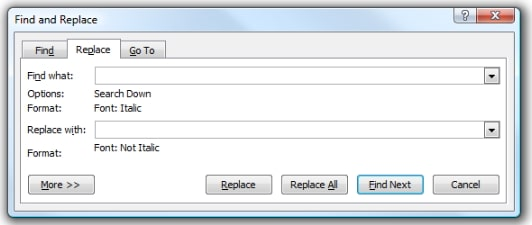### 2. Issue of Extra Space or Spaces

Similar to the previous issue of cell formatting, if you have extracted the data using CSV file format, then most probably, you will face another issue of unwanted extra spaces. You will have to face this issue mostly in the case of using VLOOKUP function of MS excel. That is used to lookup and retrieve data in a table from a specific table. With lookup columns to the right, lookup values must appear in the first column of the table. This function supports exact matching and approximate and wildcards for partial matches. It is hard to identify and remove these extra unwanted spaces. For example, if you want to use the VLOOKUP function to lookup the student name as a base, and if in your case you have two students with similar names. . Such as given in the table below.

 Simon Toffel 12 Simon Toffel 13

They look exactly the same to our eyes, but there are two blank spaces in the first name. Now using VLOOKUP, you will not get the expected output as it does not meet the criteria of the function VLOOKUP and you will get an error. You can easily fix this issue by using a Trim Formula, that will find more than one spaces between the words and will delete it. So it is always recommended to use Trim Formula before using VLOOKUP.

### 3. Workbook is Set to Manual Calculation

If you face an issue where a selection of formulas is correct but displays incorrect results that do not agree to the inputs or otherwise don't make any sense. This issue occurs when your workbook is set to manual calculation mode and thus the formulas will not be updated automatically when there is an amendment in workbook and needs to be manually forced to do so. Moreover, if you will copy and paste formula the result will be copied instead of the correct answer, unless you use the manual recalculation prompt.

To fix this issue, you can turn on the calculation back to automatic. To do so, press the Calculate button in the left corner of excel or press F9. Other way to switch the workbook back to automatic calculation is to go to Formulasà Calculation option.### 4. Hidden Characters and Non-Printable Characters

Similar to previous issues, it is also a probable cause for Excel formula not working. If your data contains such characters that are hidden or nonprintable, it is recommended to fix it before using Excel formulas.

To fix this issue, you can use a CLEAN function that is similar to the Trim Formula. It can be used to delete unwanted hidden characters,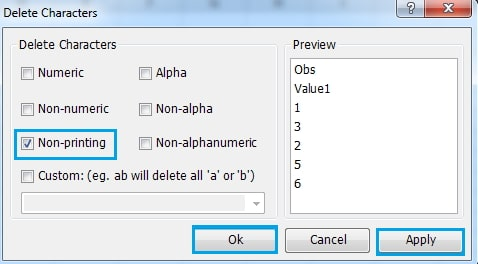### 5. Excel Is Just Showing the Formula and Not Calculating the Value

In Excel, there is an option to show all the workbook formulas. Although it is very useful to use this option, you need to take a little care when using this "Show Formulas Option", as you may not get the values for the applied formulas.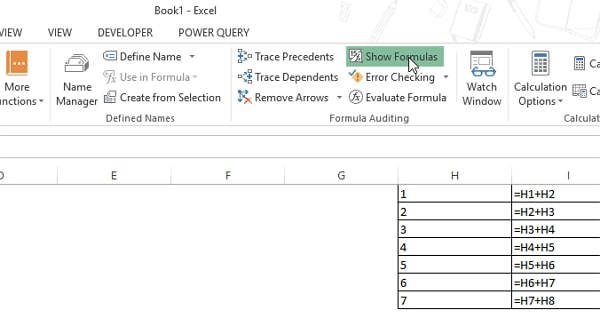### 6. Using Double Quotes Incorrectly

While writing formulas, you often use double quotes, but it is essential to know when you should use a double quotes and when not to use. As in the case of joining two numbers, you can use "12&34" to get 1234. On the other hand, you cannot use the same method to join two strings (text). You need to use double quotes. For example, =ABC&DEF will give you name error, you should use "ABC "&" DEF" to make it work correctly.

### 7. Incorrect Use of "Absolute" Referencing

Using the option of absolute referencing, you can fix the ranges of the formula to copy from one cell to another. You should take care when using this option, as it is one of the main reasons for Excel formulas not working when you copy from a cell to another cell.

To fix this issue, you need to manually analyze the formulas, as there is no quick fix for this error. Best practice is to cross check your formulas and results when you use absolute and relative referencing.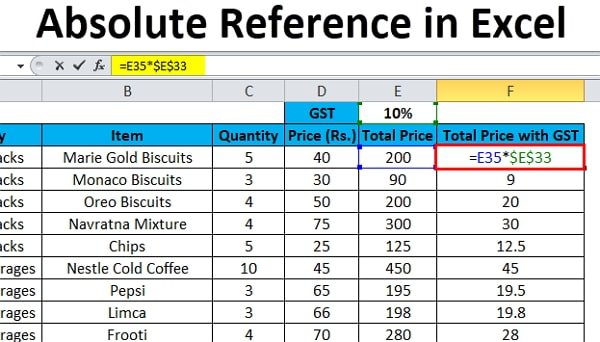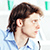By Ronnie Barnes to Excel

Posted on Mar 20, 2023 ( Updated: Mar 20, 2023)

Ronnie Barnes, a blogger with more than 5-year experience in writing tips about password recovery for Windows and office files.